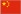简体中文

# 图解十六种折弯展开长度的计算公式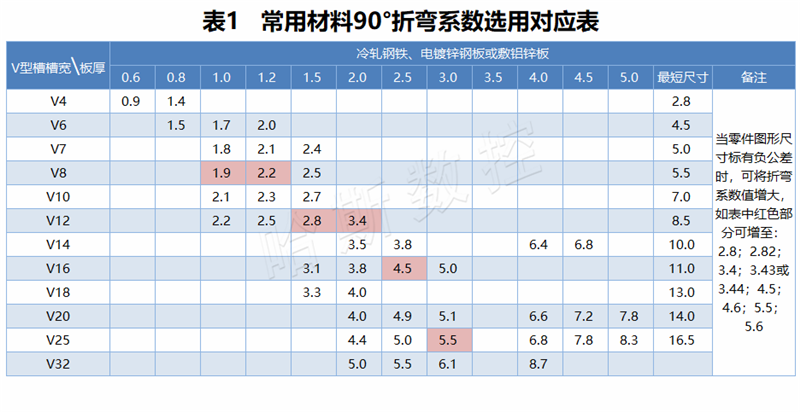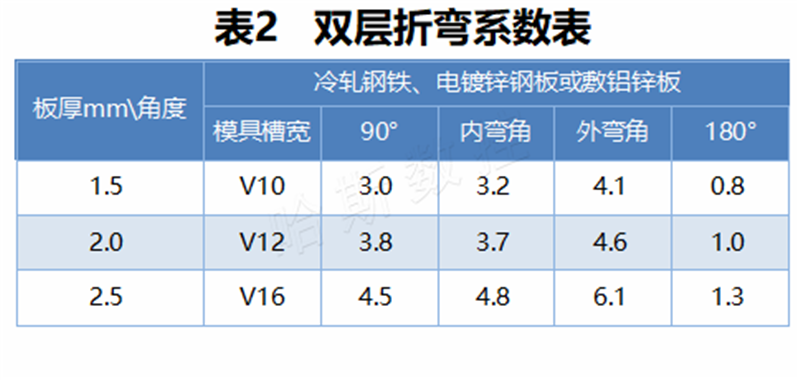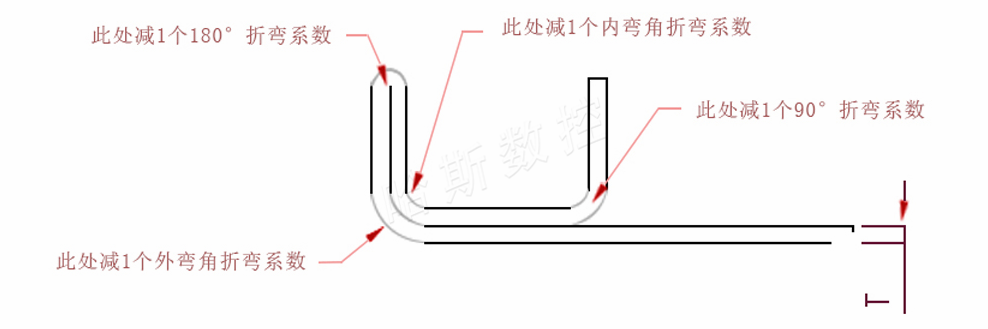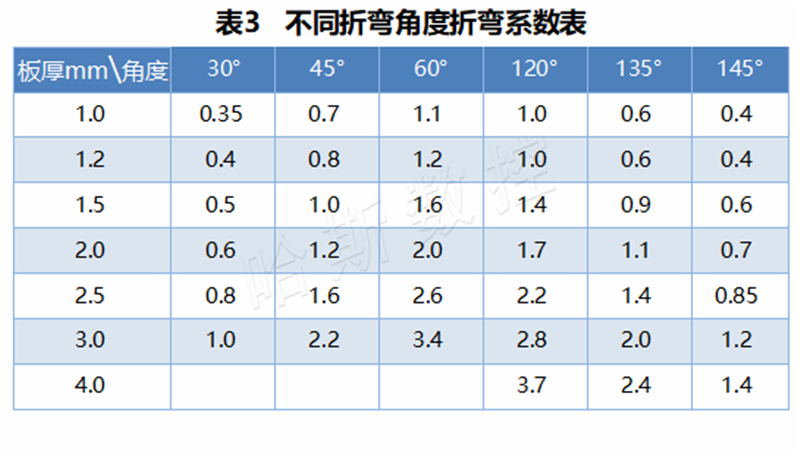A、B---工件弯边长度

P---弯边折弯系数（折弯系数：弯一刀减一个系数）

R---弯边圆角（一般为板厚）

T---材料厚度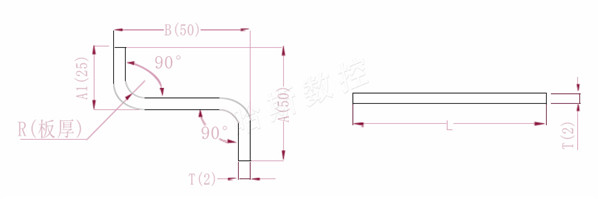A(A1)B---工件弯边长度

P---弯边折弯系数（折弯系数：弯一刀减一个系数）

R---弯边圆角（一般为板厚）

T---材料厚度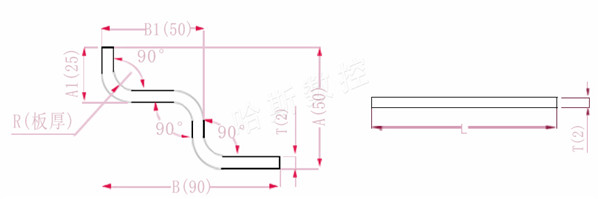A(A1)BB1---工件弯边长度

P---弯边折弯系数（折弯系数：弯一刀减一个系数）

R---弯边圆角（一般为板厚）

T---材料厚度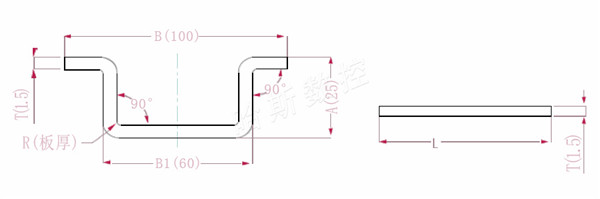ABB1---工件弯边长度

P---弯边折弯系数（折弯系数：弯一刀减一个系数）

R---弯边圆角（一般为板厚）

T---材料厚度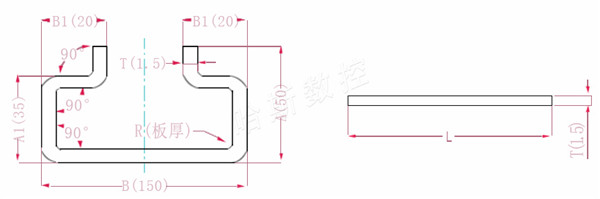AA1）、BB1---工件弯边长度

P---弯边折弯系数（折弯系数：弯一刀减一个系数）

R---弯边圆角（一般为板厚）

T---材料厚度

L=50+1.5+50+1.5+150+20+20-6*2.8=276.2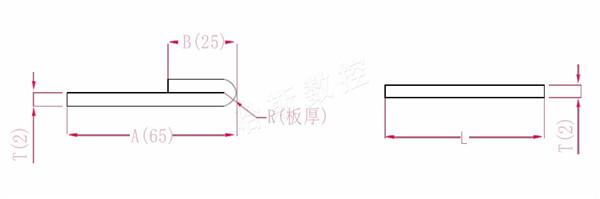AB---工件弯边长度

P---打平圆角折弯系数

R---弯边圆角（一般为板厚）

T---材料厚度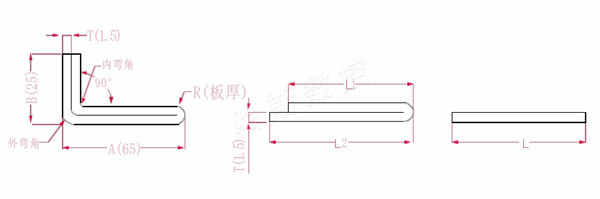AB---工件弯边长度

P1---内弯角折弯系数

P2---外弯角折弯系数

R---弯边圆角（一般为板厚）

T---材料厚度

L2=A+B-P2L2=65+25-4.1=85.9

L=L1+L2-T/2L=83.8+85.9-0.75=168.95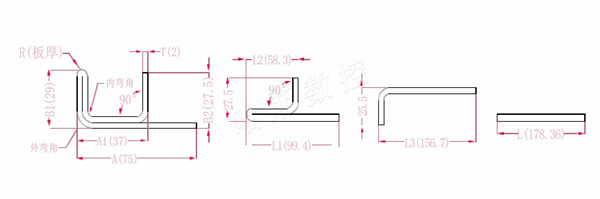AA1）、B1B2---工件弯边长度

P1---内弯角折弯系数

P2---外弯角折弯系数

P3---90°折弯系数

R---弯边圆角（一般为板厚）

T---材料厚度

P4---180°折弯系数

L2=A1-T+B1-T-P1L2=37-2+29-2-3.7=58.3

L3=L1+L2-P3L3=99.4+58.3-1=156.7

L=25.5+L3-P1L=25.5+156.7-3.84=178.36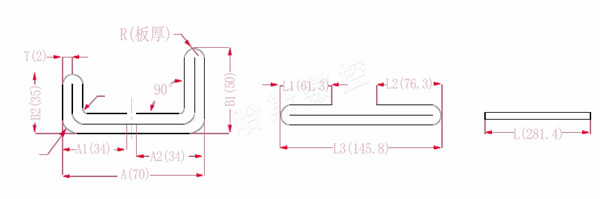AA1A2B1B2LL1L2L3---工件弯边长度

P1---内弯角折弯系数

P2---外弯角折弯系数

R---弯边圆角（一般为板厚）

T---材料厚度

L2=B1-T+A2-T-P1L2=50-2+34-2-3.7=76.3

L3=A+B1+B2-2*P2L3=70+35+50-2*4.6+145.8

L=L1+L2+L3-2*P3L=61.3+75.3+145.8-2*1=280.4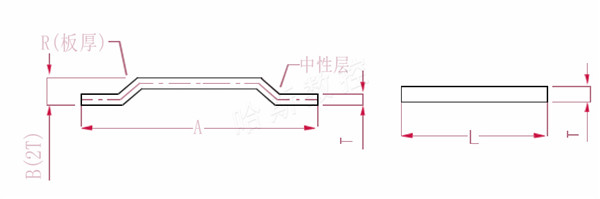AB---工件弯边长度

R---弯边圆角（一般为板厚）

T---材料厚度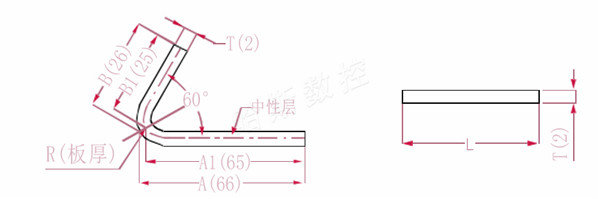AA1）、BB1---工件弯边长度

P---弯边折弯系数（折弯系数：弯一刀减一个系数）

R---弯边圆角（一般为板厚）

T---材料厚度

L=66-1+26-1-2=65+25-2=88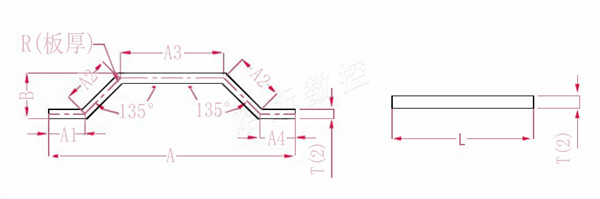AA1A2A3A4）、B---工件弯边长度

P---弯角135°折弯系数

R---弯边圆角（一般为板厚）

T---材料厚度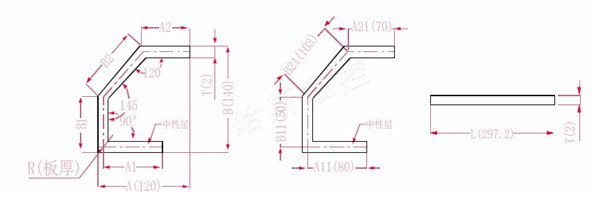AA1A2）、BB1B2---工件弯边长度

P1---120°折弯系数

P2---145°折弯系数

P3---90°折弯系数

R---弯边圆角（一般为板厚）

T---材料厚度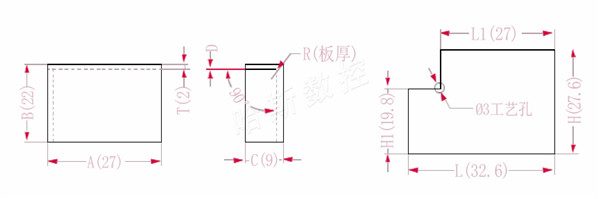ABC---工件弯边长、宽、高

P---折弯系数

R---弯边圆角（一般为板厚）

HH1）、LL1---各边展开长度

T---材料厚度

D---折弯工艺间隙（一般为0~0.5

L=A+C-P L=27+9-3.4=32.6

H1=B-T-D H1=22-2-0.2=19.8 注：D0.2

H=B+C-P H=22+9-3.4=27.6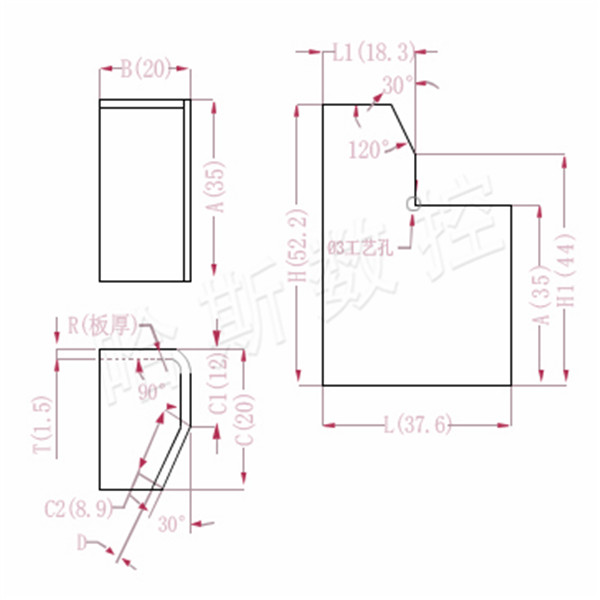ABC---工件弯边长、宽、高

HH1）、LL1---各边展开长度

P---90°折弯系数 P1---30°折弯系数

R---弯边圆角（一般为板厚）

T---材料厚度

D---折弯工艺间隙（一般为0~0.5

L=B+C1+C2-P-P1 L=20+12+8.9-2.8-0.5=37.6

H1=C1+A-P-D H1=12+35-2.8-0.2=44 注：D0.2

H=A+C-P H=35+20-2.8=52.2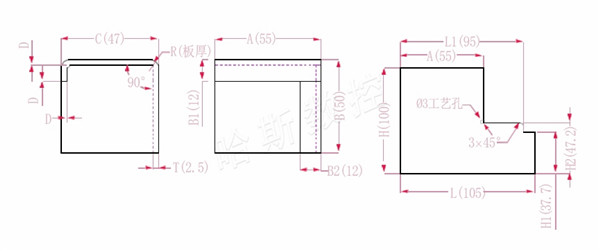ABC---工件弯边长、宽、高

HH1）、LL1---各边展开长度

P---折弯系数

R---弯边圆角（一般为板厚）

T---材料厚度

D---折弯工艺间隙（一般为0~0.5

H2=B-T-D H2=50-2.5-0.3=47.2

H=B+C+B1-2*P H=50+47+12-2*4.5=100

L1=A+C-T-D-P L1=55+47-2.5-0.3-4.5=94.7

L=A+C+B2-2*P L=55+47+12-2*4.5=105

## 联系信息

电话: 025-57269018
 邮箱: info@hasscnc.cn

地址: 江苏省南京市溧水区明觉工业园

## 邮件订阅

第一时间了解我们的剪板机和折弯机产品信息